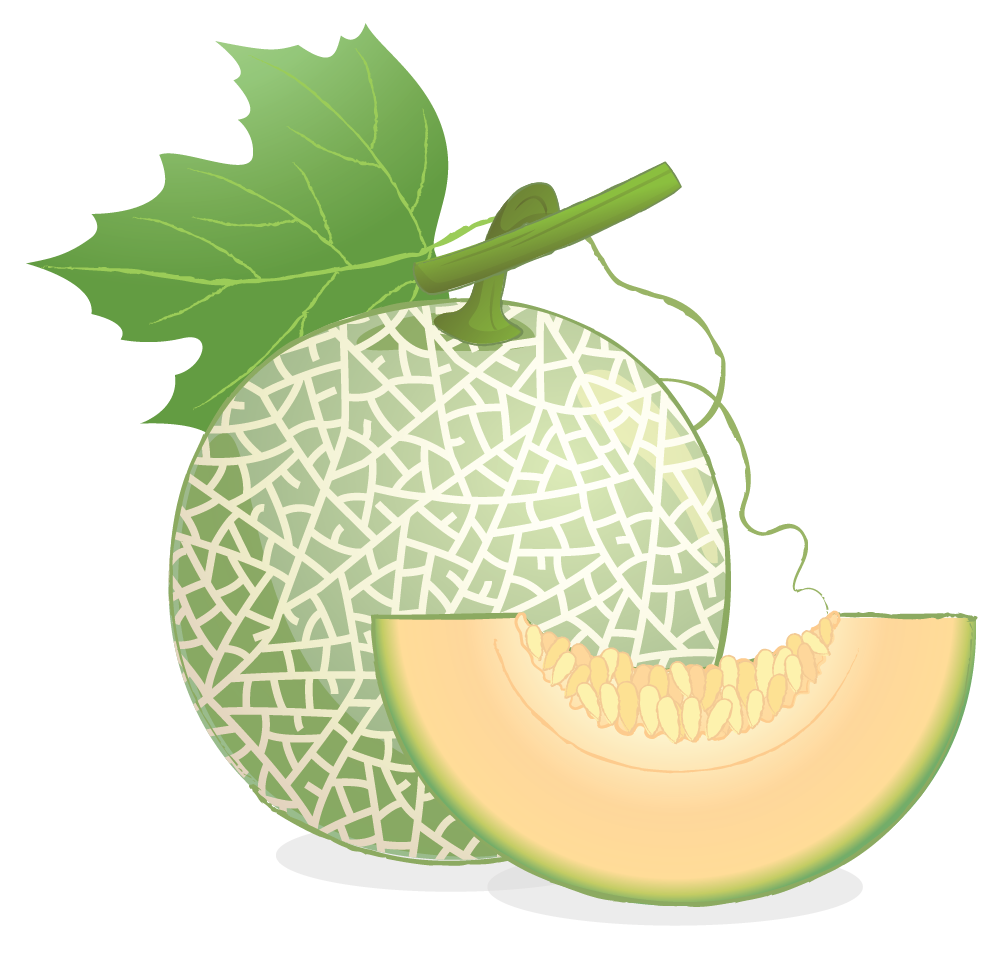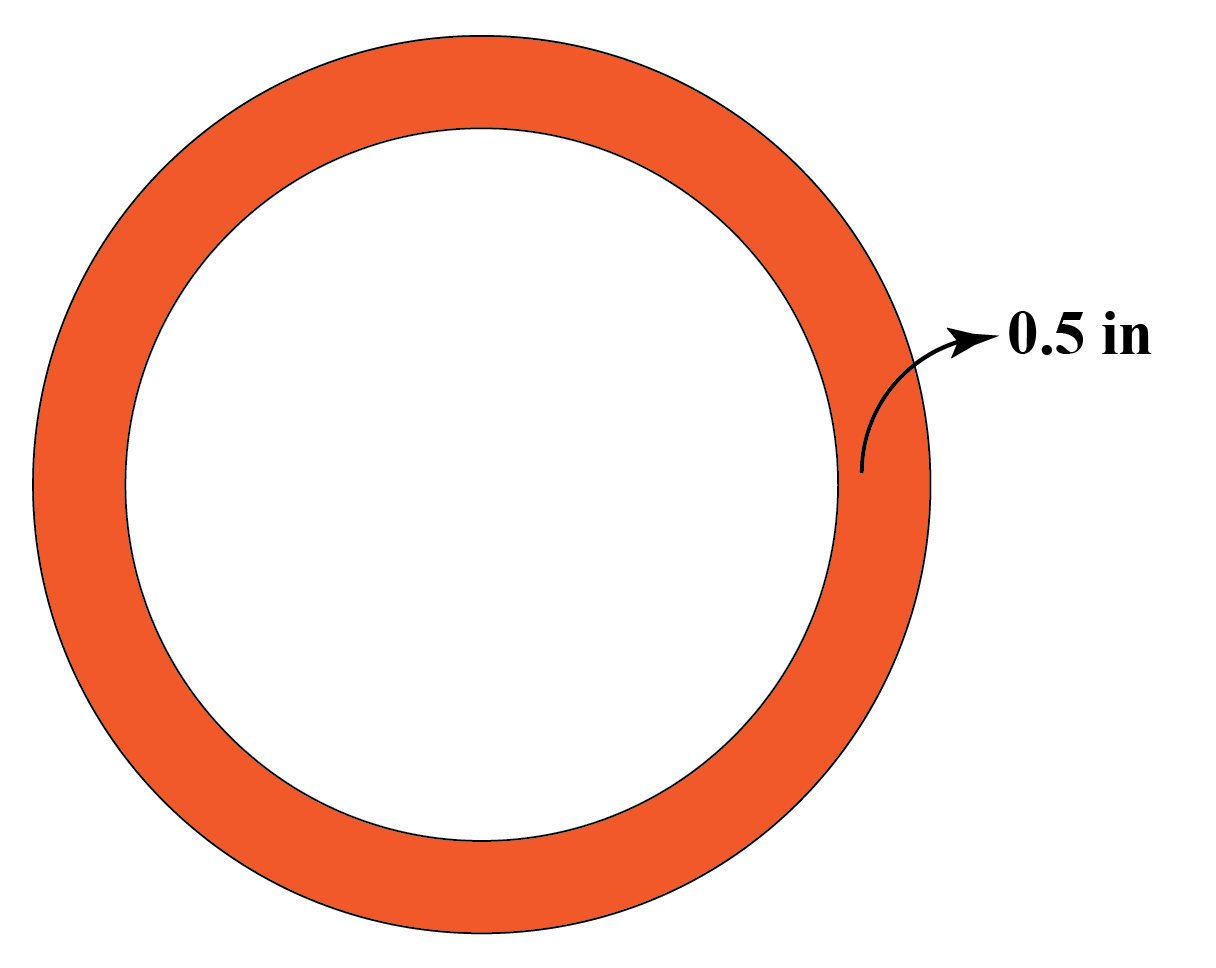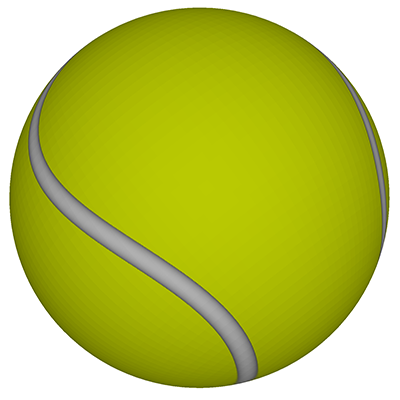# Area of Sphere

Area of Sphere
Go back to  'Area'

Marcus wants to gift a basketball to his best friend, Glen, for his birthday. He needs wrapping paper for the gift. To determine the size of the wrapping paper, he needs to know the area of the basketball.

An understanding of the surface area of a sphere will definitely help him solve this problem!

Let’s learn more about area of sphere, the volume of sphere, the circumference of a sphere and total surface area of sphere and explore surface area of sphere calculator for better understanding of the concept.

Check-out the interactive simulations to know more about the lesson and try your hand at solving a few interactive questions at the end of the page.

## What is Area of Sphere?

The surface area of a sphere refers to the region covered by its outer surface.

A sphere is a three-dimensional solid object which has a round structure, like a circle.

The difference between a sphere and a circle is that a circle is 2-dimensional, whereas, a sphere is a 3-dimensional shape.

Drag the screen and observe the surface area of the sphere in the simulation shown below.

Look at the following simulation to check the formation sphere.

Click on semicircle to know how the sphere is formed

## What is the General Formula for Area of Sphere?

The general formula for the area of a sphere is:

 Area of a Sphere = $$4 \pi r^2$$

For any three-dimensional shapes, the area of the object can be classified into three types:
•    Lateral surface area
•    Curved surface area
•    Total surface area

Lateral surface area is the area of all flat surfaces which are perpendicular to the base.
Curved surface area is the area of all the curved surfaces of the solid.
Total surface area is the area of all sides curved or lateral on the basis of the solid shapes.

As there is no flat surface in a sphere, therefore,

Curved surface area of sphere = Total surface area of a sphere

Examples of spheres:

1. All the planets in our solar system
2. Cricket ball
3. Soccer ball

## How to Calculate Area of Sphere?

The surface area of a sphere can be calculated using the formula, $$\text{A} = 4\pi r^2$$.

This formula was discovered over two thousand years ago by the Greek philosopher, Archimedes.

He also realized that the surface area of a sphere is exactly equal to the area of the curved wall of its circumscribed cylinder, which is the smallest cylinder that can contain the sphere.

Now let's try using the surface area of the sphere calculator shown below.

Enter the radius of the sphere and observe how the area is calculated.

## Solved Examples

 Example 1

A plane passes through the center of a sphere and forms a circle, with a radius of 14 feet.

What is the surface area of the sphere?

Solution

Radius = $$14 \text{ ft}$$

\begin{align}\text{Surface area of sphere} &= 4\pi r^2 \0.2cm] &= 4 \pi \times14\times14 \\[0.2cm] &= 4 \times \frac{{22}}{7} \times196 \\[0.2cm] &= 88 \times28\\[0.2cm] &= 2464 \text{ sq. ft }\\ \end{align}  $$\therefore$$ Surface area of sphere = 2464 sq.ft  Example 2 The radius of a sphere is 4.5 inches. What is the surface area of the sphere? Solution Given: Radius of the sphere (r) = 4.5 inches \begin{align} \text{Surface area of the sphere} &= 4\pi r^2 \\[0.2cm] &= 4 \pi \times (4.5)^2 \\[0.2cm] &= 4 \pi \times (\frac{9}{2})^2 \\[0.2cm] & = 4\pi \times \frac{{81}}{4} \\[0.2cm] &= 81\pi \ sq. in \\ \end{align}  $$\therefore$$ Surface area of sphere = 81$$\pi$$ sq. inches  Example 3 A cantaloupe has a diameter of about 28 inches. What would be its surface area?Solution Diameter = $$28 \text{ inches}$$ Radius = $$\frac{{28}}{2} =14 \text{ inches}$$ \begin{align} \text{Surface area of sphere } &= 4\pi r^2 \\[0.2cm] &= 4 \times \frac{{22}}{7} \times 196 \\[0.2cm] &= 88 \times28\\[0.2cm] &= 2464 \text{ sq.inches } \\ \end{align}  $$\therefore$$ Surface area of cantaloupe = 2464 sq.inches  Example 4 The cross-section of a rubber ball has an outer diameter of 22 inches. The thickness of the rubber is 0.5 inches. What is the area of the inside surface of the ball to the nearest sq. inches?Solution Outer Diameter = 22 inches Therefore, Outer Radius = 11 inches Thickness = 0.5 inches Inner Radius = Outer Radius - Thickness = 11 - 0.5 = 10.5 inches \begin{align} \text{Inside Surface area } &= 4\pi r^2 \\[0.2cm] &= 4 \pi \times \frac{21}2 \times \frac{21}2 \\[0.2cm] &= 1386 \text{ sq. inches }\\ \end{align}  $$\therefore$$ Inner surface area of the ball = 1386 sq.inches  Example 5 A spherical ball has a surface area of 2464 square inches. Find the radius of the ball.Solution \[\begin{align} \text{Surface area }&= 4\pi r^2 \\[0.2cm] \ 2464& = 4\pi r^2 \\[0.2cm] \ 2464 &= 4 \times \frac{{22}}{7} \times r^2 \\[0.2cm] \frac{{2464 \times 7}}{{4 \times 22}} &= r^2 \\[0.2cm] 196 &= r^2 \\[0.2cm] r &= 14 \\ \end{align}

 $$\therefore$$ The radius of spherical ball is 14 inchesChallenging Questions
• A sphere is cut into two equal halves and both the halves are painted from the sides. The radius of the sphere is "r" units and the rate of painting is \$5 per sq. unit. What is the total cost of painting the two halves of the sphere in dollars?
• The surface area of a sphere is 616 square inches. If its area gets reduced by 75%, what will be its new radius?

## Interactive Questions

Here are a few activities for you to practice.

## Let's Summarize

We hope you enjoyed learning about the Area of the Sphere with the simulations and practice questions. Now you will be able to easily use the surface area of sphere calculator, solve problems on volume of a sphere, the circumference of a sphere, and total surface area of the sphere.

At Cuemath, our team of math experts is dedicated to making learning fun for our favorite readers, the students!

Through an interactive and engaging learning-teaching-learning approach, the teachers explore all angles of a topic.

Be it worksheets, online classes, doubt sessions, or any other form of relation, it’s the logical thinking and smart learning approach that we, at Cuemath, believe in.

## 1. What is radius of a sphere?

A straight line segment extending from the center of a circle or sphere to the surface of the sphere.

The radius of a sphere can be calculated as, $$r = \sqrt {\frac{A}{{4\pi }}}$$

## 2. How to find the area of half sphere?

The area of half sphere or hemi-sphere can be calculated as  $$A = 3\pi r^2$$

## 3. What is CSA of sphere?

Curved surface area of a sphere is  $$A = 4\pi r^2$$

## 4. Why does the sphere have the least surface area?

The sphere has the least surface area because each point on the surface of a sphere is at an equal distance (equal to radius) from the center.

This results in a minimum surface area for a given volume.

More Important Topics
Numbers
Algebra
Geometry
Measurement
Money
Data
Trigonometry
Calculus